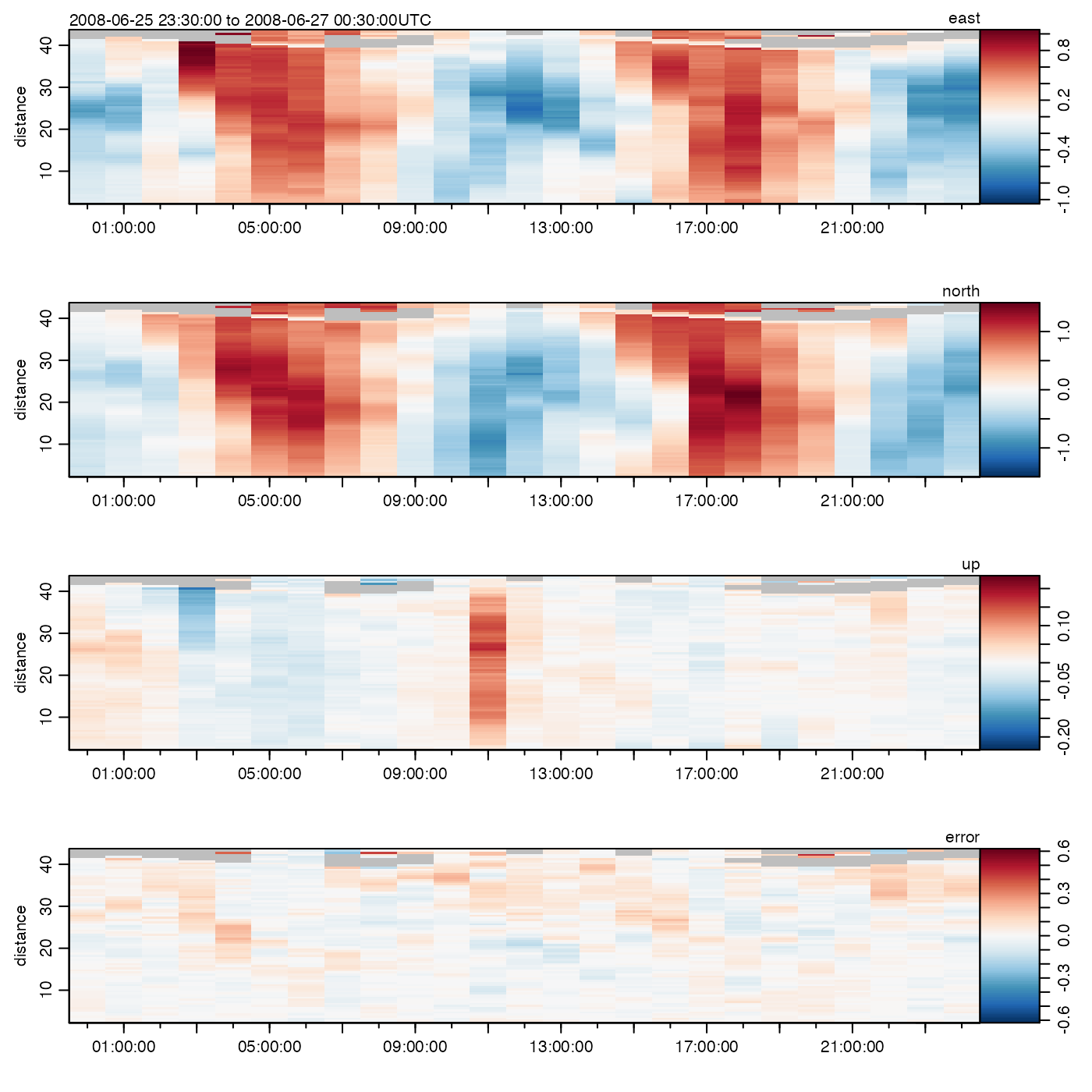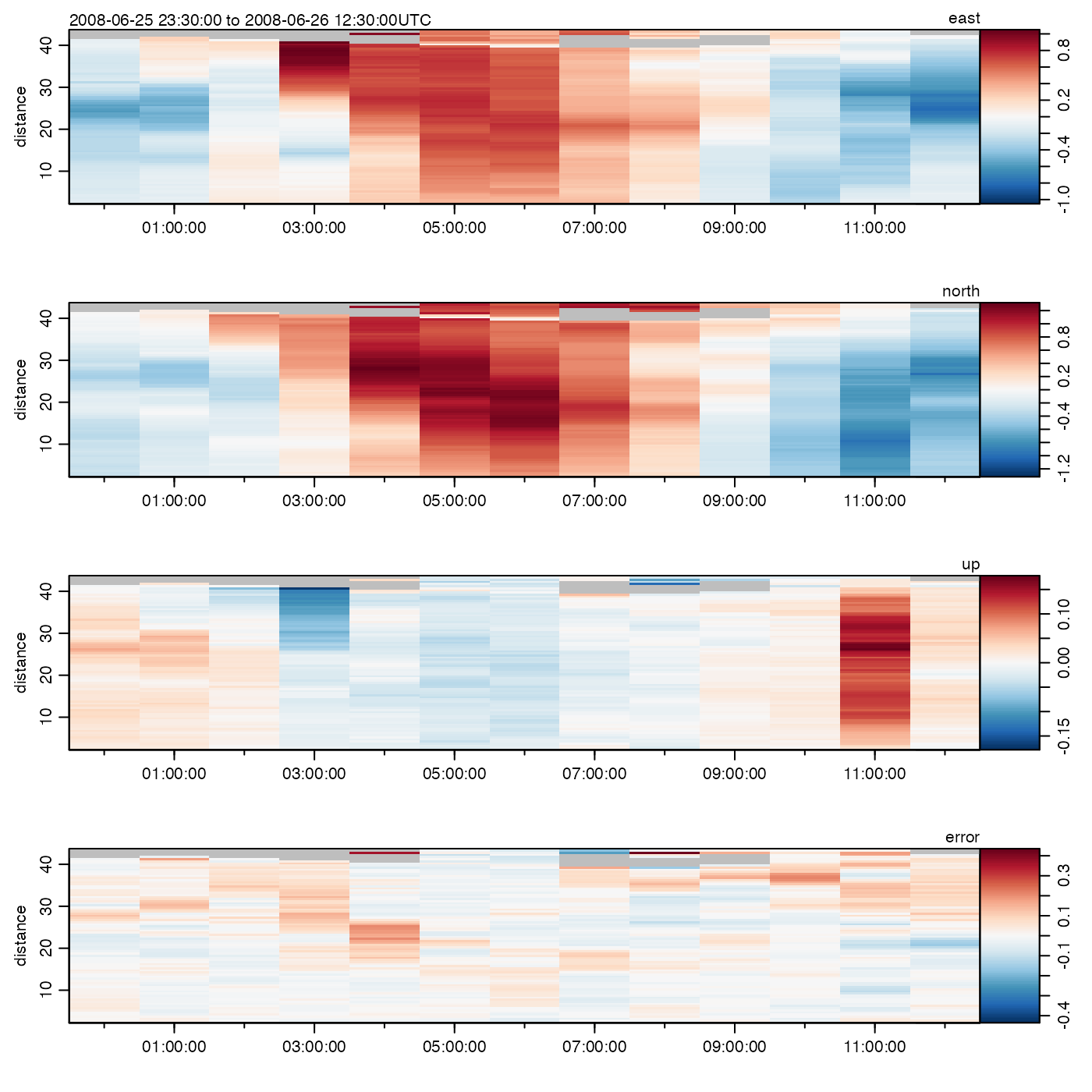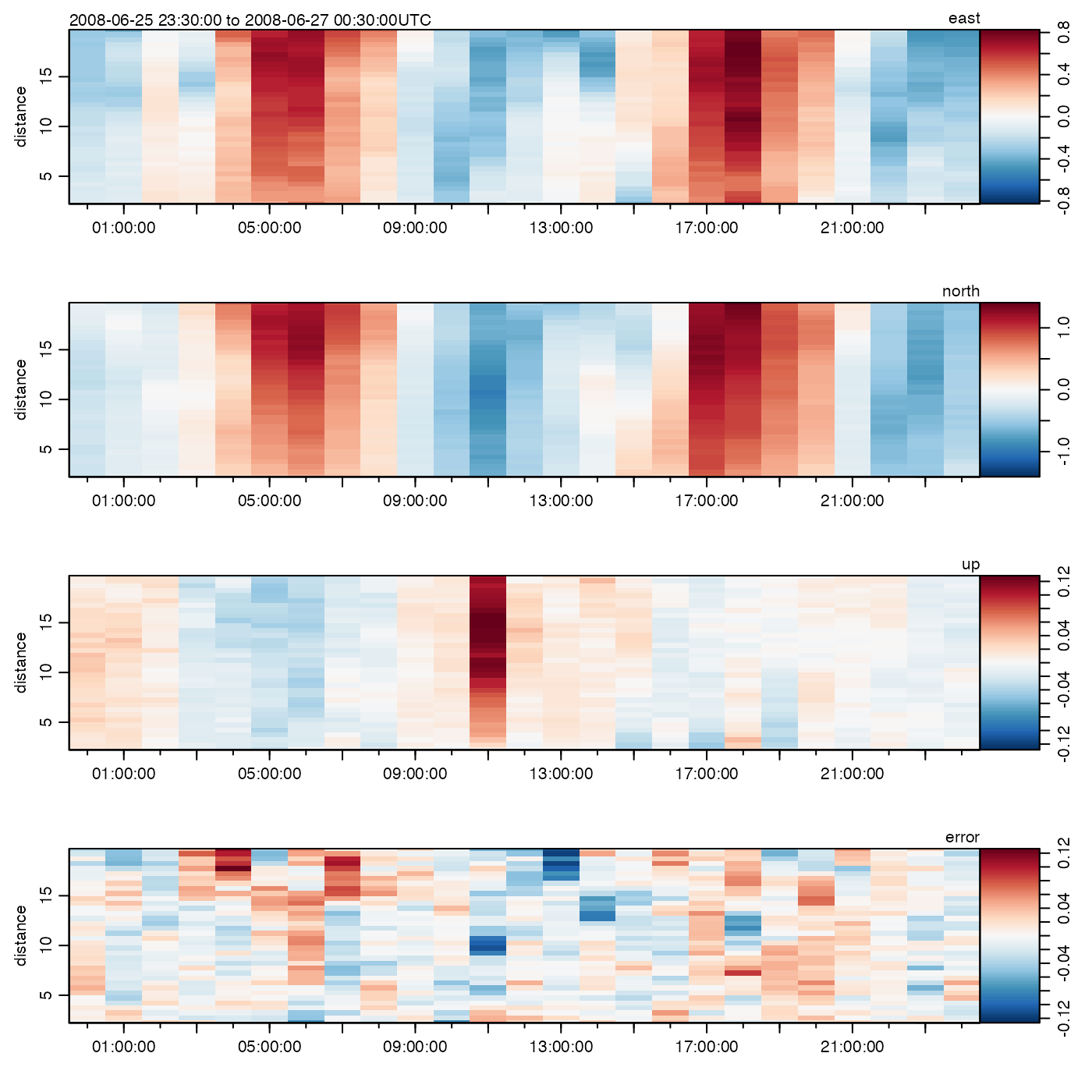Windows x on either time or distance, depending on the value of which. In each case, values of start and end may be integers, to indicate a portion of the time or distance range. If which is "time", then the start and end values may also be provided as POSIX times, or character strings indicating times (in time zone given by the value of getOption("oceTz")). Note that subset() may be more useful than this function.

# S3 method for oce
window(
x,
start = NULL,
end = NULL,
frequency = NULL,
deltat = NULL,
extend = FALSE,
which = c("time", "distance"),
indexReturn = FALSE,
debug = getOption("oceDebug"),
...
)

## Arguments

x an oce object. the start time (or distance) of the time (or space) region of interest. This may be a single value or a vector. the end time (or distance) of the time (or space) region of interest. This may be a single value or a vector. not permitted yet. not permitted yet not permitted yet string containing the name of the quantity on which sampling is done. Possibilities are "time", which applies the windowing on the time entry of the data slot, and "distance", for the distance entry (for those objects, such as adp, that have this entry). boolean flag indicating whether to return a list of the "kept" indices for the time entry of the data slot, as well as the timeSlow entry, if there is one.. Either of these lists will be NULL, if the object lacks the relevant items. a flag that turns on debugging. ignored

## Value

Normally, this is new oce object. However, if indexReturn=TRUE, the return value is two-element list containing items named index and indexSlow, which are the indices for the time entry of the data slot (and the timeSlow, if it exists).

subset() provides more flexible selection of subsets.
library(oce)
plot(adp)early <- window(adp, start="2008-06-26 00:00:00", end="2008-06-26 12:00:00")
plot(early)bottom <- window(adp, start=0, end=20, which="distance")
plot(bottom)